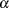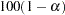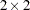The SURVEYFREQ Procedure
 TABLES Statement

TABLES requests </ options> ;

The TABLES statement requests one-way to n-way frequency and crosstabulation tables and statistics for these tables.

If you omit the TABLES statement, PROC SURVEYFREQ generates one-way frequency tables for all DATA= data set variables that are not listed in the other statements.

The following argument is required in the TABLES statement:

requests

specify the frequency and crosstabulation tables to produce. A request is composed of one variable name or several variable names separated by asterisks. To request a one-way frequency table, use a single variable. To request a two-way crosstabulation table, use an asterisk between two variables. To request a multiway table (an n-way table, where n>2), separate the desired variables with asterisks. The unique values of these variables form the rows, columns, and layers of the table.

For two-way tables to multiway tables, the values of the last variable form the crosstabulation table columns, while the values of the next-to-last variable form the rows. Each level (or combination of levels) of the other variables forms one layer. PROC SURVEYFREQ produces a separate crosstabulation table for each layer. For example, a specification of A*B*C*D in a TABLES statement produces k tables, where k is the number of different combinations of levels for A and B. Each table lists the levels for D (columns) within each level of C (rows).

You can use multiple TABLES statements in a single PROC SURVEYFREQ step. You can also specify any number of table requests in a single TABLES statement. To specify multiple table requests quickly, use a grouping syntax by placing parentheses around several variables and joining other variables or variable combinations. Table 83.2 shows some examples of grouping syntax.

Table 83.2 Grouping Syntax

Request

Equivalent to

tables A*(B C);

tables A*B    A*C;

tables (A B)*(C D);

tables A*C    B*C    A*D    B*D;

tables (A B C)*D;

tables A*D    B*D    C*D;

tables A – – C;

tables A    B    C;

tables (A – – C)*D;

tables A*D    B*D    C*D;

The TABLES statement variables are one or more variables from the DATA= input data set. These variables can be either character or numeric, but the procedure treats them as categorical variables. PROC SURVEYFREQ uses the formatted values of the TABLES variable to determine the categorical variable levels. So if you assign a format to a variable with a FORMAT statement, PROC SURVEYFREQ formats the values before dividing observations into the levels of a frequency or crosstabulation table. See the discussion of the FORMAT procedure in the Base SAS Procedures Guide and the discussions of the FORMAT statement and SAS formats in SAS Language Reference: Dictionary.

The frequency or crosstabulation table lists the values of both character and numeric variables in ascending order based on internal (unformatted) variable values unless you change the order with the ORDER= option. To list the values in ascending order by formatted value, use ORDER=FORMATTED in the PROC SURVEYFREQ statement.

Without Options

If you request a frequency or crosstabulation table without specifying options, PROC SURVEYFREQ produces the following for each table level or cell:

• frequency, or sample size

• weighted frequency, which estimates the population total

• standard deviation of the weighted frequency

• percentage, which estimates the population proportion

• standard error of the percentage

The table displays weighted frequencies if your analysis includes a WEIGHT statement, or if you specify the WTFREQ option in the TABLES statement. The table also displays the number of observations with missing values. See the sections One-Way Frequency Tables and Crosstabulation Tables for more information.

Options

Table 83.3 lists the options available in the TABLES statement. Descriptions follow in alphabetical order.

Table 83.3 TABLES Statement Options

Option

Description

Control Statistical Analysis

sets the level for confidence limits

requests Rao-Scott chi-square test

requests Rao-Scott modified chi-square test

specifies degrees of freedom

requests Rao-Scott likelihood ratio test

requests Rao-Scott modified likelihood ratio test

requests odds ratio and relative risks

requests risks and risk difference

specifies null proportions for one-way chi-square test

requests Wald chi-square test

requests Wald log-linear chi-square test

displays confidence limits for percentages

displays confidence limits for weighted frequencies

displays column percentages and standard errors

displays coefficients of variation for percentages

displays coefficients of variation for weighted frequencies

displays design effects for percentages

displays expected weighted frequencies (two-way tables)

displays row percentages and standard errors

displays variances for percentages

displays variances for weighted frequencies

displays totals and standard errors

when there is no WEIGHT statement

Control Displayed Output

suppresses display of overall percentages

suppresses display of frequency counts

suppresses display of all percentages

suppresses display of tables but displays statistical tests

suppresses display of zero rows and columns

suppresses display of standard errors for all estimates

suppresses display of row and column totals

suppresses display of weighted frequencies

You can specify the following options in a TABLES statement.

ALPHA=sets the level for confidence limits. The value ofmust be between 0 and 1, and the default is 0.05. A confidence level ofproduces% confidence limits. The default of ALPHA=0.05 produces 95% confidence limits.

You request confidence limits for percentages with the CL option, and you request confidence limits for weighted frequencies with the CLWT option. See the section Confidence Limits for more information.

The ALPHA= option also applies to confidence limits for the risks and risk difference, which you request with the RISK option, and to confidence limits for the odds ratio and relative risks, which you request with the OR option. See the sections Risks and Risk Difference and Odds Ratio and Relative Risks for details.

CHISQ

requests the Rao-Scott chi-square test. This test applies a design effect correction to the Pearson chi-square statistic computed from the weighted frequencies. See the section Rao-Scott Chi-Square Test for details.

By default for one-way tables, the CHISQ option provides a design-based goodness-of-fit test for equal proportions. To compute the test for other null hypothesis proportions, specify the null proportions with the TESTP= option.

The CHISQ option uses proportion estimates to compute the design effect correction. To use null hypothesis proportions instead, specify the CHISQ1 option.

CHISQ1

requests the Rao-Scott modified chi-square test. This test applies a design effect correction to the Pearson chi-square statistic computed from the weighted frequencies, and bases the design effect correction on null hypothesis proportions. See the section Rao-Scott Chi-Square Test for details. To compute the design effect correction from proportion estimates instead of null proportions, specify the CHISQ option.

By default for one-way tables, the CHISQ option provides a design-based goodness-of-fit test for equal proportions. To compute the test for other null hypothesis proportions, specify the null proportions with the TESTP= option.

CL

requests confidence limits for the percentages, or proportions, in the crosstabulation table. PROC SURVEYFREQ determines the confidence coefficient from the ALPHA= option, which by default equals 0.05 and produces 95% confidence limits. See the section Confidence Limits for more information.

CLWT

requests confidence limits for the weighted frequencies, or totals, in the crosstabulation table. PROC SURVEYFREQ determines the confidence coefficient from the ALPHA= option, which by default equals 0.05 and produces 95% confidence limits. See the section Confidence Limits for more information.

COL

displays the column percentage, or estimated proportion of the column total, for each cell in a two-way table. The COL option also provides the standard errors of the column percentages. See the section Row and Column Proportions for more information. This option has no effect for one-way tables.

CV

displays the coefficient of variation for each percentage, or proportion estimate, in the crosstabulation table. See the section Coefficient of Variation for more information.

CVWT

displays the coefficient of variation for each weighted frequency, or estimated total, in the crosstabulation table. See the section Coefficient of Variation for more information.

DEFF

displays the design effect for each overall proportion estimate in the crosstabulation table. See the section Design Effect for more information.

DF=df

specifies the degrees of freedom for the analysis. The value of df must be a nonnegative number. By default, PROC SURVEYFREQ computes the degrees of freedom as described in the section Degrees of Freedom.

PROC SURVEYFREQ uses the DF= value in computing confidence limits for proportions, totals, and other statistics. See the section Confidence Limits for details. PROC SURVEYFREQ also uses the DF= value in computing the denominator degrees of freedom for the F statistics in the Rao-Scott and Wald chi-square tests. See the sections Rao-Scott Chi-Square Test, Rao-Scott Likelihood Ratio Chi-Square Test, Wald Chi-Square Test, and Wald Log-Linear Chi-Square Test for more information.

EXPECTED

displays expected weighted frequencies for the table cells in a two-way table. The expected frequencies are computed under the null hypothesis that the row and column variables are independent. See the section Expected Weighted Frequency for more information. This option has no effect for one-way tables.

LRCHISQ

requests the Rao-Scott likelihood ratio chi-square test. This test applies a design effect correction to the likelihood ratio chi-square statistic computed from the weighted frequencies. See the section Rao-Scott Likelihood Ratio Chi-Square Test for more information.

By default for one-way tables, the LRCHISQ option provides a design-based test for equal proportions. To compute the test for other null hypothesis proportions, specify the null proportions with the TESTP= option.

The LRCHISQ option uses proportion estimates to compute the design effect correction. To use null hypothesis proportions instead, specify the LRCHISQ1 option.

LRCHISQ1

requests the Rao-Scott modified likelihood ratio chi-square test. This test applies a design effect correction to the likelihood ratio chi-square statistic computed from the weighted frequencies, and bases the design effect correction on null hypothesis proportions. See the section Rao-Scott Likelihood Ratio Chi-Square Test for more information. To compute the design effect correction from proportion estimates instead of null proportions, specify the LRCHISQ option.

By default for one-way tables, the LRCHISQ option provides a design-based test for equal proportions. To compute the test for other null hypothesis proportions, specify the null proportions with the TESTP= option.

NOCELLPERCENT

suppresses the display of overall cell percentages in the crosstabulation table, as well as the standard errors of the percentages. The NOCELLPERCENT option does not suppress the display of row or column percentages, which you request with the ROW or COL option.

NOFREQ

suppresses the display of cell frequencies in the crosstabulation table. The NOFREQ option also suppresses the display of row, column, and overall table frequencies.

NOPERCENT

suppresses the display of all percentages in the crosstabulation table. The NOPERCENT option also suppresses the display of standard errors of the percentages. Use the NOCELLPERCENT option to suppress display of overall cell percentages but allow display of row or column percentages.

NOPRINT

suppresses the display of frequency and crosstabulation tables but displays all requested statistical tests. Note that this option disables the Output Delivery System (ODS) for the suppressed tables. For more information, see Chapter 20, Using the Output Delivery System.

NOSPARSE

suppresses the display of variable levels with zero frequency in two-way tables. By default, the procedure displays all levels of the column variable within each level of the row variable, including any column variable levels with zero frequency for that row. For multiway tables, the procedure displays all levels of the row variable for each layer of the table by default, including any row variable levels with zero frequency for the layer.

NOSTD

suppresses the display of all standard errors in the crosstabulation table.

NOTOTAL

suppresses the display of row totals, column totals, and overall totals in the crosstabulation table.

NOWT

suppresses the display of weighted frequencies in the crosstabulation table. The NOWT option also suppresses the display of standard errors of the weighted frequencies.

OR

requests estimates of the odds ratio, the column 1 relative risk, and the column 2 relative risk fortables. The OR option also provides confidence limits for these statistics. See the section Odds Ratio and Relative Risks for details.

To compute confidence limits for the odds ratio and relative risks, PROC SURVEYFREQ determines the confidence coefficient from the ALPHA= option, which by default equals 0.05 and produces 95% confidence limits.

RISK

requests risk statistics fortables. The RISK option also provides standard errors and confidence limits for these statistics. Risk statistics include the row 1 risk (or proportion), row 2 risk, overall risk, and risk difference. See the section Risks and Risk Difference for details.

The RISK option provides both column 1 and column 2 risks. To request only column 1 or column 2 risks, use the RISK1 or RISK2 option.

To compute confidence limits for the risks and risk difference, PROC SURVEYFREQ determines the confidence coefficient from the ALPHA= option, which by default equals 0.05 and produces 95% confidence limits.

RISK1

requests column 1 risk statistics fortables, together with their standard errors and confidence limits. Risk statistics include the row 1 risk (or proportion), row 2 risk, overall risk, and risk difference. See the section Risks and Risk Difference for details.

To compute confidence limits for the risks and risk difference, PROC SURVEYFREQ determines the confidence coefficient from the ALPHA= option, which by default equals 0.05 and produces 95% confidence limits.

RISK2

requests column 2 risk statistics fortables, together with their standard errors and confidence limits. Risk statistics include the row 1 risk (or proportion), row 2 risk, overall risk, and risk difference. See the section Risks and Risk Difference for details.

To compute confidence limits for the risks and risk difference, PROC SURVEYFREQ determines the confidence coefficient from the ALPHA= option, which by default equals 0.05 and produces 95% confidence limits.

ROW

displays the row percentage, or estimated proportion of the row total, for each cell in a two-way table. The ROW option also provides the standard errors of the row percentages. See the section Row and Column Proportions for more information. This option has no effect for one-way tables.

TESTP=(values)

specifies null hypothesis proportions, or test percentages, for one-way chi-square tests. You can separate values with blanks or commas. Specify values in probability form as numbers between 0 and 1, where the proportions sum to 1. Or specify values in percentage form as numbers between 0 and 100, where the percentages sum to 100. PROC SURVEYFREQ treats the value 1 as the percentage form 1%. The number of TESTP= values must equal the number of variable levels in the one-way table. List these values in the same order in which the corresponding variable levels appear in the output.

When you specify the TESTP= option, PROC SURVEYFREQ displays the specified test percentages in the one-way frequency table. The TESTP= option has no effect for two-way tables.

PROC SURVEYFREQ uses the TESTP= values for all one-way chi-square tests you request in the TABLES statement. The available one-way chi-square tests include the Rao-Scott (Pearson) chi-square test and the Rao-Scott likelihood ratio chi-square test and their modified versions, which you request with the CHISQ, CHISQ1, LRCHISQ, and LRCHISQ1 options. See the sections Rao-Scott Chi-Square Test and Rao-Scott Likelihood Ratio Chi-Square Test for details.

VAR

displays the variance estimate for each percentage in the crosstabulation table. See the section Proportions for details. By default, PROC SURVEYFREQ displays the standard errors of the percentages.

VARWT

displays the variance estimate for each weighted frequency, or estimated total, in the crosstabulation table. See the section Totals for details. By default, PROC SURVEYFREQ displays the standard deviations of the weighted frequencies.

WCHISQ

requests the Wald chi-square test for two-way tables. See the section Wald Chi-Square Test for details.

WLLCHISQ

requests the Wald log-linear chi-square test for two-way tables. See the section Wald Log-Linear Chi-Square Test for details.

WTFREQ

displays totals (weighted frequencies) and their standard errors when you do not specify a WEIGHT or REPWEIGHTS statement. PROC SURVEYFREQ displays the weighted frequencies by default when you include a WEIGHT or REPWEIGHTS statement. Without a WEIGHT or REPWEIGHTS statement, PROC SURVEYFREQ assigns all observations a weight of one.Previous Page | Next Page | Top of Page